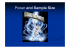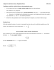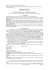# Typical Problem One Sample Estimation

## Transcription

Typical Problem One Sample Estimation
```Typical Problem
One Sample Estimation
Bret Larget
The following data set are the weights (mg) of thymus glands from
five chick embryos after 14 days of incubation.
The data was collected as part of a study on development of the
thymus gland.
> thymus
Departments of Botany and of Statistics
Statistics 371
14th October 2005
Statistical Estimation
 29.6 21.5 28.0 34.6 44.9
If we model this data as having been sampled at random from a
population of chick embryos with similar conditions, what can we
say about the population mean weight?
Standard Error of the Mean
I
I
Statistical inference is inference about unknown aspects of a
population based on treating the observed data as the
realization of a random process.
I
We focus in this course on inference in the setting of random
samples from populations.
I
Statistical estimation is a form of statistical inference in which
we use the data to estimate a feature of the population and to
assess the precision the estimate.
I
Chapter 6 introduces these ideas in the setting of estimating a
population mean µ.
We know that SD of the sampling distribution of the sample
mean y¯ can be computed by this formula.
σ
σY¯ = √
n
I
I
I
But if we only observe sample data y1 , . . . , yn , we do not
know the value of the population SD σ, so we cannot use the
formula directly.
However, we can compute the sample standard deviation s,
which is an estimate of the population standard deviation σ.
The expression
s
SEY¯ = √
n
is called the standard error of the sample mean and is an
estimate of the standard deviation of the sampling distribution
of the sample mean. (You can understand why statisticians
gave this concept a shorter name.)
Example (cont.)
I
Here is some R code to compute the mean, standard
deviation, and standard error for the example data.
> m = mean(thymus)
> m
 31.72
> s = sd(thymus)
> s
 8.72909
> n = length(thymus)
> n
 5
Derivation of a Confidence Interval
From the sampling distribution of Y¯ , we have the following
statement
½
¾
σ
σ
P µ − z √ ≤ Y¯ ≤ µ + z √
= 0.9
n
n
if we let z = 1.645, because the area between −1.645 and 1.645
under a standard normal curve is 0.9. Different choices of z work
for different confidence levels.
The first inequality is equivalent to
σ
µ ≤ Y¯ + z √
n
> se = s/sqrt(n)
> se
 3.903767
I
I
The sample standard deviation is an estimate of how far
individual values differ from the population mean.
and the second is equivalent to
σ
Y¯ − z √ ≤ µ
n
The standard error is an estimate of how far sample means
from samples of size n differ from the population mean.
Confidence intervals
The basic idea of a confidence interval for µ is as follows.
I
We know that the sample mean y¯ is likely to be close (within
√
a few multiples of σ/ n) to the population mean µ.
I
Thus, the unknown population mean µ is likely to be close to
the observed sample mean y¯ .
I
We can express a confidence interval by centering an interval
around the observed sample mean y¯ — those are the possible
values of µ that would be most likely to produce a sample
mean y¯ .
Derivation (cont.)
We put these together to give
¾
½
σ
σ
¯
¯
= 0.9
P Y −z√ ≤ µ ≤ Y +z√
n
n
This recipe for a confidence interval is then
σ
Y¯ ± z √
n
I
This depends on knowing σ.
I
If we don’t know σ as is usually the case, we could use s as an
alternative.
I
However, the probability statement is then no longer true.
I
We need to use a different multiplier to account for the extra
uncertainty.
I
This multiplier comes from the t distribution.
Sampling Distributions
The t Distributions in R
y¯ − µ
Z = ±√
σ n
I
The functions pt and qt find areas and quantiles of t
distributions in R.
I
The area to the right of 2.13 under a t distribution with 4
degrees of freedom is
y¯ − µ
T = ±√
s n
I
If the population is normal, the statistic Z has a standard
normal distribution.
I
If the population is not normal but n is sufficiently large, the
statistic Z has approximately a standard normal distribution
(by the Central Limit Theorem).
I
I
> 1 - pt(2.27, 4)
 0.04286382
I
> qt(0.95, df = 4)
 2.131847
I
The distribution of the statistic T is more variable than that
of Z because there is extra randomness in the denominator.
The extra randomness becomes small as the sample size n
increases.
To find the 95th percentile of the t distribution with four
degrees of freedom, you could do the following.
This R code cecks the values of the 0.05 upper tail probability
for the first several rows of the table.
> round(qt(0.95, df = 1:10), 3)
 6.314 2.920 2.353 2.132 2.015 1.943 1.895 1.860 1.833 1.812
I
You can use R to find values not tabulated.
> qt(0.95, 77)
 1.664885
Student’s t Distribution
I
Mechanics of a confidence interval
If Y1 , . . . , Yn are a random sample from any normal
distribution and if Y¯ and S are the sample mean and standard
deviation, respectively, then the statistic
I
Y¯ − µ
T = ±√
S
n
I
I
I
is said to have a t distribution with n − 1 degrees of freedom.
All t distributions are symmetric, bell-shaped, distributions
centered at 0, but their shapes are not quite the same as
normal curves and they are spread out a more than the
standard normal curve.
The spread is largest for small sample sizes. As the sample
size (and degrees of freedom) increases, the t distributions
become closer to the standard normal distribution.
The Table in the back cover of your textbook provides a few
key quantiles for several different t distributions.
A confidence interval for µ takes on the form
s
Y¯ ± t × √
n
where t is selected so that the area between −t and t under a
t distribution curve with n − 1 degrees of freedom is the
desired confidence level.
I
In the example, there are df = n − 1 = 4 degrees of freedom.
I
A 90% confidence interval uses the multiplier t = 2.132.
I
A 95% confidence interval would use t = 2.776 instead.
Mechanics (cont.)
We are 90% confident that the mean thymus weight in
the population is in the interval 31.72 ± 8.32 or
(23.4, 40.04).
We are 95% confident that the mean thymus weight in
the population is in the interval 31.72 ± 10.84 or
(20.88, 42.56).
I
Notice that these multipliers 2.132 and 2.776 are each greater
than the corresponding z multipliers 1.645 and 1.96.
I
Had the sample size been 50 instead of 5, the t multipiers
1.677 and 2.01 would still be larger than the corresponding z,
but by a much smaller amount.
Interpretation of a confidence interval
In our real data example, we would interpret the 90% confidence
interval as follows.
We are 90% confident that the mean thymus weight (mg)
of all similar chick embyos that had been incubated under
similar conditions would be between 23.4 and 40.04.
Another Example
The diameter of a wheat plant is an important trait because it is
related to stem breakage which affects harvest. The stem
diameters (mm) of a sample of eight soft red winter wheat plants
taken three weeks after flowering are below.
2.3
states the confidence level;
I
states the parameter being estimated;
I
is in the context of the problem, including units; and
I
describes the population.
It is generally good practice to round the margin of error to two
significant figures and then round the estimate to the same
precision.
2.4
2.2
2.3
2.5
1.9
2.0
The mean and standard deviation are y¯ = 2.275 and s = 0.238.
(a) Find a 95% confidence interval for the population mean.
(b) Interpret the confidence interval in the context of the problem.
True or False
I
True or False. From the same data, a 99% confidence interval
would be larger.
I
True or False. In a second sample of size eight, there is a 95%
probability that the sample mean will be within 0.20 of 2.28.
I
True or False. In a second sample of size eight, there is a 95%
probability that the sample mean will be within 0.20 of the
population mean µ.
I
True or False. The probability is 95% that the sample mean is
between 2.08 and 2.48.
I
True or False. In the population, 95% of all individuals have
stem diameters between 2.08 and 2.48 mm.
I
True or False. We can be 95% confident that 95% of all
individuals in the population have stem diameters between
2.08 and 2.48 mm.
Notice that the interpretation of a confidence interval
I
2.6
How big should n be?
I
I
Confidence Intervals for Proportions
When planning a study, you may want to know how large a
sample size needs to be so that your standard error is at least
as small as a given size.
Guessed SD
√
n
µ
Guessed SD
Desired SE
¶2
Conditions for Validity
I
I
I
I
I
I
The mean and standard deviation are 1/n times the mean and
SD of a binomial distribution.
Namley, the mean is p and the
q
standard deviation is
I
After solving for n, we have this.
n=
I
The sampling distribution of pˆ is the shape of a binomial
distribution, but is on the values 0, 1/n, 2/n, . . . , 1 instead of
the integers 0, 1, 2, . . . , n.
Solving this problem is a matter of plugging in a guess for the
population SD and solving for n.
Desired SE =
I
I
The most important condition is that the sampling process be
like simple random sampling.
If the sampling process is biased, the confidence interval will
greatly overstate the true confidence we should have that the
confidence interval contains µ.
If we have random sampling from a non-normal population,
the confidence intervals are approximately valid if n is large
enough so that the sampling distribution of Y¯ is
approximately normal.
The answer to this question depends on the degree of
non-normality.
Specifically, strongly skewed distributions require large n for
the approximations to be good.
Non-normal population shapes that are nonetheless symmetric
converge to normal looking sampling distributions for
relatively small n.
I
p(1−p)
.
n
The conventional 95% confidence interval for p plugs in the
estimate pˆ for p in the formula for the standard error.
r
pˆ(1 − pˆ)
pˆ ± 1.96
n
This formula has been shown to behave erratically in the sense
that the actual probability of containing p fluctuates with n
and is often less than 95%. The size of the error decreases
only slowly and erratically with increases in n.
Better Method
I
An alternative method is to compute p˜, the sample proportion
from a ficiticious sample with four more observations, two
successes and two failures.
I
If pˆ = y /n, then p˜ = (y + 2)/(n + 4). The SE calculated as
r
p˜(1 − p˜)
SEp˜ =
n
produce better overall confidence intervals.
A 95% confidence interval for p is computed with the formula
p˜ ± 1.96SEp˜
Note that this confidence interval is not centered at the
sample proportion, but rather is sampled at an adjusted
proportion. This method is actually a Bayesian method in
disguise as a frequentist method.
```

### Small-Sample C.I.s for one- sample, two-sample and (matched) paired data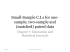### SAMPLE MEANS 8/4/2014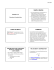### 1.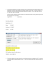### Lecture 3 Research Methods Lecture 10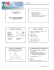### Document 6540260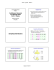### Confidence intervals for sample means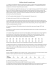### Chapter 7  μ Confidence Intervals and Sample Size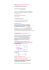### MODUL STATISTIKA UNTUK BISNIS DAN MANAJEMEN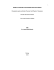### MATH 140 SEMESTER REVIEW PROBLEMS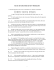### Math 121 - Sample Final Exam - F07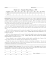### Power and Sample Size